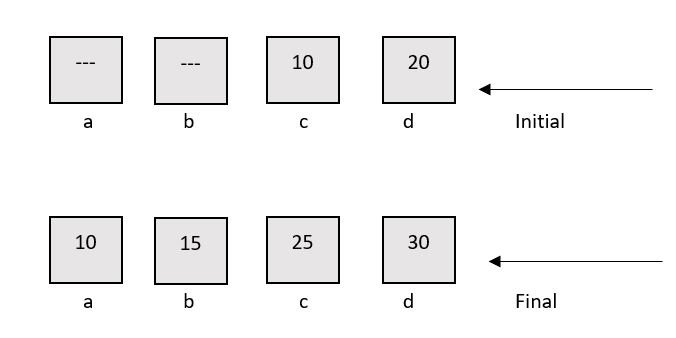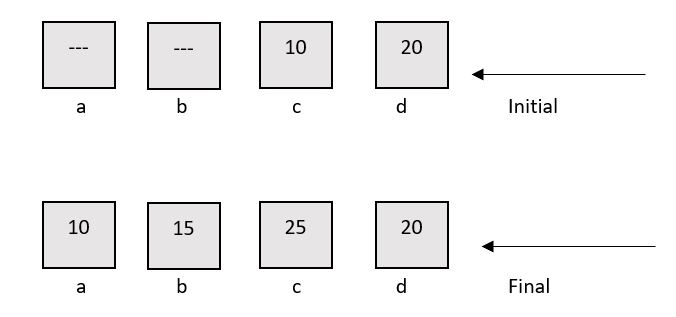×

Search anything:

# Default arguments in C++

#### C++ Software Engineering function default argumentsGet this book -> Problems on Array: For Interviews and Competitive Programming

A default argument is a value provided in a function declaration that is automatically assigned by the compiler if the caller of the function doesn’t provide a value for the argument with a default value.

Syntax :

``````data_type fun_name(data_type1 var1 = value1)
``````

### Example

``````#include<iostream>
using namespace std;

// A function with default arguments, it can be called with
// 2 arguments or 3 arguments or 4 arguments.
int sum(int a, int b, int c=10, int d=20)
{
return (a+b+c+d);
}

/* Driver program to test above function*/
int main()
{
cout << sum(10, 15) << endl;
cout << sum(10, 15, 25) << endl;
cout << sum(10, 15, 25, 30) << endl;
return 0;
}
``````

Output:

``````55
70
80
``````

### Points to remeber

1. Default arguments are different from constant arguments as constant arguments can’t be changed whereas default arguments can be overwritten if required.

Example :

``````int sum(int a, int b, int c=10, int d=20)
{
return (a+b+c+d);
}
sum(10, 15, 25, 30)
``````1. Default arguments are overwritten when calling function provides values for them. For example, calling of function sum(10, 15, 25, 30) overwrites the value of z and w to 25 and 30 respectively.

Example :1. During calling of function, arguments from calling function to called function are copied from left to right. Therefore, sum(10, 15, 25) will assign 10, 15 and 25 to x, y, and z. Therefore, the default value is used for w only.

Example :

``````int sum(int a, int b, int c=10, int d=20)
{
return (a+b+c+d);
}
sum(10, 15, 25)
``````4)Once default value is used for an argument in function definition, all subsequent arguments to it must have default value.
It can also be stated as default arguments are assigned from right to left. For example, the following function definition is invalid as subsequent argument of default variable z is not default.

Example :

``````// Invalid because z has default value, but w after it
// doesn't have default value
int sum(int x, int y, int z=10, int w)
``````

### Question

Consider this code statement:

``````int sum(int a=10, int b=20, int c);
``````

#### Is the above C++ statement valid?

No
Yes
Logical error
Syntax error
As mentioned in the article since a has default value assigned, all the arguments after a (in this case b and c) must have default values assigned, b has default value but c doesn't have, thats why this is also invalid.#### Harshita Sahai

Maintainer at OpenGenus | Previously Software Developer, Intern at OpenGenus (June to August 2019) | B.Tech in Information Technology from Guru Gobind Singh Indraprastha University (2017 to 2021)

Default arguments in C++